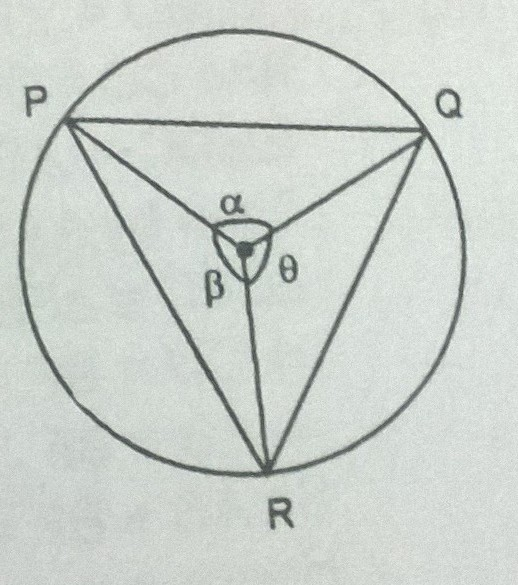Window of my soul

Geometry Level 4In the figure above, area of circle is 50 and area of triangle is 15.

If the value of $\sin\theta + \sin\alpha + \sin\beta$ equal to $\dfrac mn \pi$ for coprime positive integers $m$ and $n$, find the value of $m+n$.

×